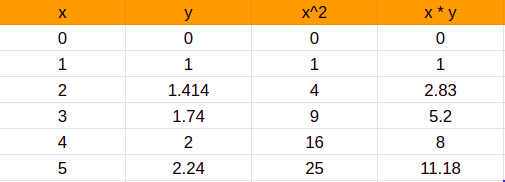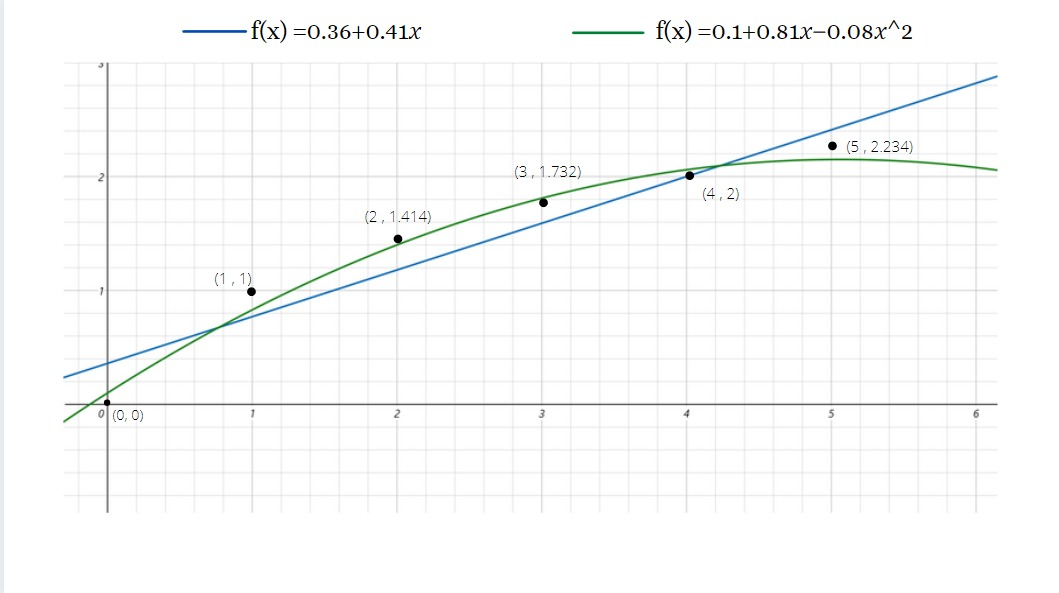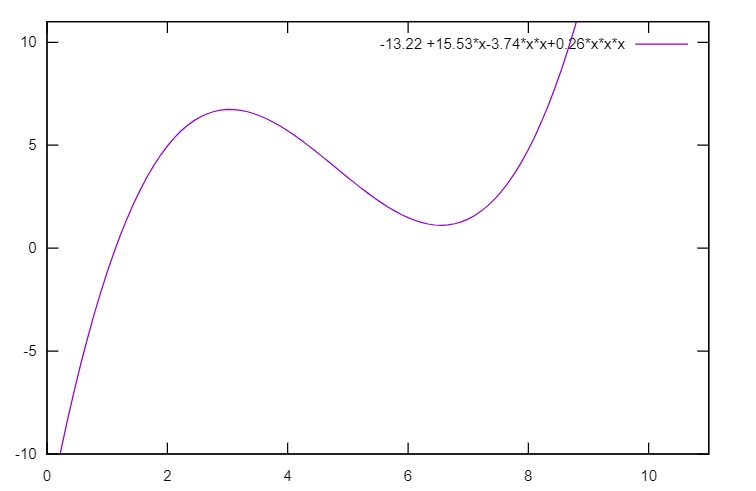Least squares curve fitting is one of the  basic and commonly used method for curve fitting .

suppose we have a data set of points$(x_1,y_1) ,(x_2,y_2) , ..... ,(x_n,y_n)$

the first step in curve fitting is to choose a promising or intended function form$f(x)$ , essentially a parameterised function ,to fit the data points ,Polynomial function form is one of the form that is used widely$f(x) = a_0 +a_1 x+ a_2 x^2 + a_3 x^3 + ... + a_n x^n$

here we have to determine the coefficients$a_0 ,a_1 ,a_2 ,a_3 ,... ,a_n$ from the above equation , so that we can find the set of these coefficients ( data points ) such that the function best fits the given data .
by using the least square method we can minimise the root-mean-square error$E(f) = \sqrt{\frac{1}{n} \sum_{i=1} ^n (\epsilon_i) ^2}$

here$\epsilon_i$ is the error at the$i ^th$ point
so ,$\epsilon_i$ is the distance between the data value$y_i$ and the fitted value$\hat y_i = f(x_i)$ on the curve$\epsilon_i = y_i - f(x_i)$

the least square error method finds the set of coordinates for$\hat a_0 ,\hat a_1 ,\hat a_2 , \hat a_3 ,... ,\hat a_n$ and minimises the root mean square of error$E(f)$ . As a result , the Fitted curve will be$f(x) = \hat a_0 +\hat a_1 x +\hat a_2 x^ + \hat a_3 x^3 ,... ,\hat a_n x^n$

 x 0 1 2 3 4 5 y 0 1 1.414 1.73 2 2.24

Now after selecting the data set ,we need to decide the function that we will use the function to fit the data .
Now let us analyse the data by a plotting them as an intuitive view of the relationship between x and y

(IN general curve-fitting of n data points , an (n-1) degree of polynomial fits best for all the data points )

Since we have taken 6 data points ,the polynomial of degree 5 ,can pass exactly through all the data points

Now lets us try to find the values of$f(x)$ for a polynomial of degree 2from the above table$\sum x = 15 \\ \\ \sum y = 8.384 \\ \\ \sum x^2=55 \\ \\ \sum x^3=225 \\ \\ \sum x^4 =979 \\ \\ \sum (x \times y ) =28.21 \\ \\ \sum (x^2 \times y) = 110.15$

The equation is$y=a+bx+cx^2$ and the normal equations are$\sum y = an +b \sum x + c \sum x^2$,$\sum xy = a \sum x + b \sum x^2 + c \sum x^3$,$\sum x^2y = a \sum x^2 + b \sum x^3 + c \sum x^4$,

Now Substituting these values in the normal equations$6a+15b+55c=8.38 ...(1)$$15a+55b+225c=28.2 .... (2)$$55a+225b+979c=110.14 ....(3)$

On solving the above 3 equations ,we get$a = 0.1 \\ b = 0.81 \\ c = -0.08 \\$
now on substitution the above values in in the equation$y = a + bx + cx^2$
we get$y = 0.1 +0.81x-0.08x^2$

				
%use s2
var data_ls = SortedOrderedPairs(
doubleArrayOf(0.0, 1.0, 2.0, 3.0, 4.0, 5.0),
doubleArrayOf(0.0, 1.0, 1.414, 1.74, 2.0, 2.24))
var ls : LeastSquares = LeastSquares(2)
// here the 2 represents the highest degree of polynomial we are using
var fls : UnivariateRealFunction = ls.fit(data_ls)
var f_0 = fls.evaluate(0.0)
var f_1 = fls.evaluate(1.0)
var f_2 = fls.evaluate(2.0)
var f_3 = fls.evaluate(3.0)
var f_4 = fls.evaluate(4.0)
var f_5 = fls.evaluate(5.0)
//the error value can be obtained by
println(String.format("f(%f) = %f and the error value is %f", 0.0, f_0, 0.0-f_0))
println(String.format("f(%f) = %f and the error value is %f", 1.0, f_1, 1.0-f_1))
println(String.format("f(%f) = %f and the error value is %f", 2.0, f_2, 1.414-f_2))
println(String.format("f(%f) = %f and the error value is %f", 3.0, f_3, 1.732-f_3))
println(String.format("f(%f) = %f and the error value is %f", 4.0, f_4, 2.0-f_4))
println(String.format("f(%f) = %f and the error value is %f", 5.0, f_5, 2.24-f_5))




Output :

f(0.000000) = 0.098571 and the error value is -0.098571
f(1.000000) = 0.829029 and the error value is 0.170971
f(2.000000) = 1.401771 and the error value is 0.012229
f(3.000000) = 1.816800 and the error value is -0.084800
f(4.000000) = 2.074114 and the error value is -0.074114
f(5.000000) = 2.173714 and the error value is 0.066286

Now let us calculate the same for the above data set for a linear equationfrom above table we get$\sum x = 15 \\ \\ \sum y = 8.384 \\ \\ \sum x^2=55 \\ \\ \sum (x \times y ) =28.21$

Now let us the least squares for the linear equation$y = a + bx$
Now the normal equations for the linear equation are$\sum y = an + b \sum x$$\sum xy = a \sum x + b \sum x^2$

now let us substitute the value in the equation$y= a + bx$$6a+15b=8.39 ....(1) \\ \\ 15a+55b=28.22 ....(2)$

On solving equations (1) and (2) we get$a = 0.36 \\ \\ b = 0.41$
here ,now the equation becomes$y = 0.36 +0.41x$

				
%use s2
var data_ls = SortedOrderedPairs(
doubleArrayOf(0.0, 1.0, 2.0, 3.0, 4.0, 5.0),
doubleArrayOf(0.0, 1.0, 1.414, 1.74, 2.0, 2.24))
var ls : LeastSquares = LeastSquares(1)
// here the 2 represents the highest degree of polynomial we are using
var fls : UnivariateRealFunction = ls.fit(data_ls)
var f_0 = fls.evaluate(0.0)
var f_1 = fls.evaluate(1.0)
var f_2 = fls.evaluate(2.0)
var f_3 = fls.evaluate(3.0)
var f_4 = fls.evaluate(4.0)
var f_5 = fls.evaluate(5.0)
//the error value can be obtained by
println(String.format("f(%f) = %f and the error value is %f", 0.0, f_0, 0.0-f_0))
println(String.format("f(%f) = %f and the error value is %f", 1.0, f_1, 1.0-f_1))
println(String.format("f(%f) = %f and the error value is %f", 2.0, f_2, 1.414-f_2))
println(String.format("f(%f) = %f and the error value is %f", 3.0, f_3, 1.732-f_3))
println(String.format("f(%f) = %f and the error value is %f", 4.0, f_4, 2.0-f_4))
println(String.format("f(%f) = %f and the error value is %f", 5.0, f_5, 2.24-f_5))




Output :

f(0.000000) = 0.361429 and the error value is -0.361429
f(1.000000) = 0.776457 and the error value is 0.223543
f(2.000000) = 1.191486 and the error value is 0.222514
f(3.000000) = 1.606514 and the error value is 0.125486
f(4.000000) = 2.021543 and the error value is -0.021543
f(5.000000) = 2.436571 and the error value is -0.196571

Now let us compare the plots for the above 2 examples

				
%use s2
// plotting the  above function using JGnuplot
val p = JGnuplot(false)
p.getXAxis().setBoundaries(-20.0, 20.0)
p.getYAxis().setBoundaries(-20.0, 20.0)
p.plot()



Output :when compared the results with the linear equation and the quadratic equation for the above data set ,the data seems to be fitted in curve of higher order polynomial when compared to the linear equation..

However, for analyzing the trend of the given data the linear equations seems to be more accurate , in the quadratic equation for the data the predicted values have a sudden fall . Thus we can say the the data can be fitted more precisely in the higher degree polynomial with less noise and linear equations are used in finding the trend of the data set given.Example : 3

now let us try for an other example with a different data set.

 x 2 4 5 8 y 5 6 5 7

from the above table

The general equations are$\sum y = an + b \sum x + c \sum x^2 + d \sum x^3$$\sum xy = a \sum x + b \sum x^2 + c \sum x^3 + d \sum x^4$$\sum x^2y = a \sum x^2 + b \sum x^3 + c \sum x^4 + d \sum x^5$$\sum x^3y = a \sum x^3 + b \sum x^4 + c \sum x^5 + d \sum x^6$

for getting a equation of degree 3 which is$y = a + bx + cx^2 + dx^3$

Now let us calculate the necessary terms for calculating the general form equationsfrom the above table we get that$4a+19b+109c+709d=22 .....(1)$$19a+109b+709c+4993d=110 ....(2)$$109a+709b+4993c+36949d=664 ....(3)$$709a+4993b+36949c+281929d=4508....(4)$

on solving the above equation we get$a=-13.22 \\ b=15.53 \\ c=-3.74 \\ d=0.26$

the final equation is$y = -13.22 +15.53x-3.74x^2+0.26x^3$

				
%use s2
var data_ls = SortedOrderedPairs(
doubleArrayOf(2.0, 4.0, 5.0, 8.0),
doubleArrayOf(5.0, 6.0, 5.0, 7.0))
var ls : LeastSquares = LeastSquares(3)
// here the 3 represents the highest degree of polynomial we are using
var fls : UnivariateRealFunction = ls.fit(data_ls)
var f_0 = fls.evaluate(2.0)
var f_1 = fls.evaluate(4.0)
var f_2 = fls.evaluate(5.0)
var f_3 = fls.evaluate(8.0)
//the error value can be obtained by
println(String.format("f(%f) = %f and the error value is %f", 2.0, f_0, 5.0-f_0))
println(String.format("f(%f) = %f and the error value is %f", 4.0, f_1, 6.0-f_1))
println(String.format("f(%f) = %f and the error value is %f", 5.0, f_2, 5.0-f_2))
println(String.format("f(%f) = %f and the error value is %f", 8.0, f_3, 7.0-f_3))



Output :

f(2.000000) = 5.000000 and the error value is 0.000000
f(4.000000) = 6.000000 and the error value is 0.000000
f(5.000000) = 5.000000 and the error value is -0.000000
f(8.000000) = 7.000000 and the error value is 0.000000
				
%use s2
// plotting the  above function using JGnuplot
val p = JGnuplot(false)
p.getXAxis().setBoundaries(0.0, 11.0)
p.getYAxis().setBoundaries(-10.0, 11.0)
p.plot()



Output :Here we can observe that the error values for the above data when calculated the (n-1)th degree equations for n data points ,the curve fits perfectly with out any noises . the corresponding values for (n-1) and (n) are given below

(n-1) = 3

n = 4